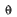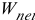# Work

Work is the transfer of energy through a distance by application of a force.

There are 2 conditions that must be met in order for work to take place:

1. a force must be applied to an object

2. the object must move in the direction of the applied force

If the force is applied at an angle, that must be taken into consideration and the component of the force which actually moves the object must be calculated.

Work = force X distance X cos Θ (where Θ is angle) (This is for work done by a constant force)

When work is done by a force applied at an angle to the direction of motion,

W = Fd cosWhen motion and force are in the same direction, Θ = 0.

Because work is the transfer of energy, it is measured in the same units in which we measure energy.   In the SI system, energy and work are measured in joules (J).

When work is done by a net force on an object and the only change in the object is its speed, the work done is equal to the change in the objects kinetic energy= KE final – KE initial

An example of this would be towing a car or boat. (Neglecting the energy lost to frictional forces.)

While work is a scalar quantity and has no direction, it is possible to do negative work.     Negative work actually means that you are losing energy out of the system. You will see this later on in Thermodynamics.

(source)